In this tutorial, we are going to learn about C arithmetic operations on integers, characters, mixed data types operators.

## 1. Arithmetic Operators – integers:

An operator is a special symbol used to manipulate data. The data items that the operators act upon are called operands.

The operator that works on a single operand is called a unary operator and that which works on two operands is known as a binary operator.

C provides many types of operators. They are:

• Arithmetic
• Unary
• Relational and equality
• Logical
• Assignment
• Conditional
• Bitwise and Special operators

In this article let’s see the Arithmetic operators in C, we have 5 arithmetic operators in C: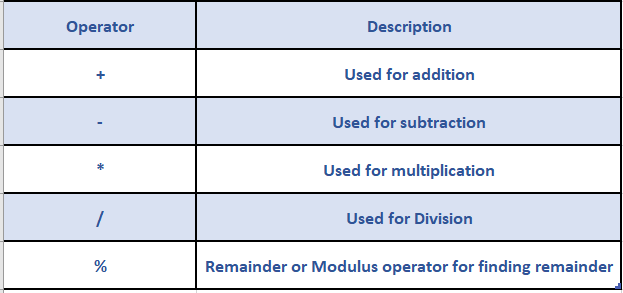Arithmetic operators are applied on numeric operands. Thus the operands can be integers, floats or characters (Since a character is internally represented by its numeric code).

The remainder operator (%) requires that both the operands be integers and the second operand be non-zero. Similarly, the division operator (/) requires that the second operand be non-zero.

The format for the usage of the arithmetic operator is as follows:

``operand1 operator operand2``

According to the coding conventions in C, a single space should be provided to the left and to the right of an operator.

The table given below demonstrates the use of various arithmetic operators using two variables p and q of type int with values 35 and 17 respectively: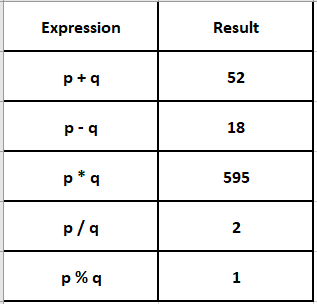## 2. Arithmetic Operators – float:

Division of one integer by another integer is referred to as integer division. This operation always results in an integer with a truncated quotient.

If a division operation is carried out with two floating-point numbers or with one floating-point number and one integer, the result will be a floating-point quotient.

The table given below demonstrates the usage of various arithmetic operators using two variables num1 and num2 of type float with values 12.5 and 2.0 respectively: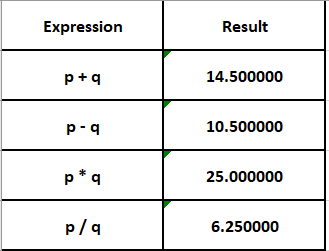Note that the remainder operator (%) is not applicable for floating-point numbers.

## 3. Arithmetic Operators – characters:

The table given below demonstrates the use of various arithmetic operators using two variables v1 and v2 of type char with values ‘Á’ and ‘D’ respectively.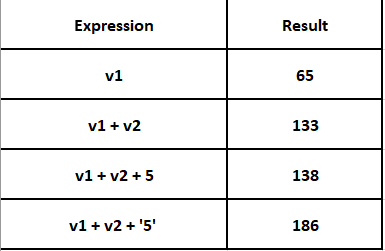In the above example, the character  ‘A’ is substituted with its ASCII value 65, and ‘D’ is substituted with 68. The character ‘5’ is substituted with its ASCII value ’53’. The integer value 5 is used as it is.

The following table demonstrates the usage of various arithmetic operators using two variables a and b of type int with values 11 and -3 respectively.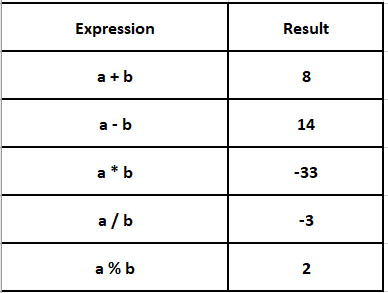## 4. Arithmetic Operators – mixed data types:

Arithmetic operators can be used in expressions with operands of different data types.

For example, the addition operator (+) can be used on int and float data types with the result being a float data type.

If the same is performed on float and double data types, the result will be a double data type, i.e., the result will always be of the data type with the highest memory capacity.

Consider the following examples with different combinations of arithmetic operators and data types.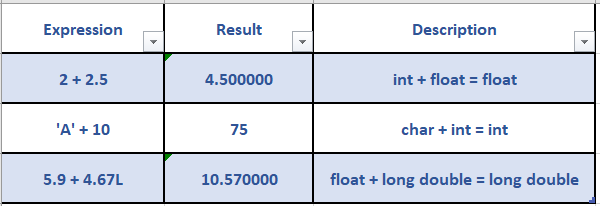Happy Learning 🙂

### About the Author: Hemanth Krishna Vineel RamayanamI’m an Undergraduate Student at the Indian Institute of Information Technology, Design, and Manufacturing, Kurnool, Andhra Pradesh. I belong to the CSE domain.